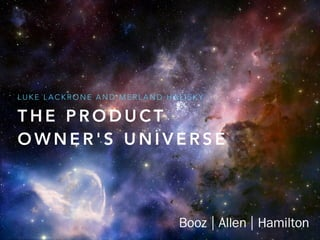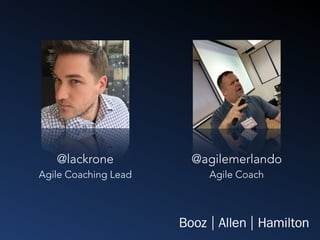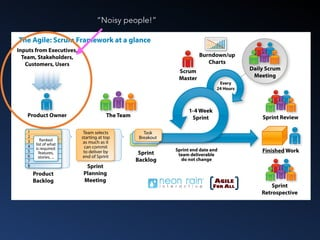Diese Präsentation wurde erfolgreich gemeldet.

# The Product Owner’s Universe: Agile Coaching

Anzeige
Anzeige
Anzeige
Anzeige
Anzeige
Anzeige
Anzeige
Anzeige
Anzeige
Anzeige
Anzeige×

1 von 13 Anzeige

# The Product Owner’s Universe: Agile Coaching

An interactive workshop that guides you through the many relationships that exist in an agile team, with a business value emphasis. Team members gain empathy, discover expectations of others and the importance of these agile team relationships.

An interactive workshop that guides you through the many relationships that exist in an agile team, with a business value emphasis. Team members gain empathy, discover expectations of others and the importance of these agile team relationships.

Anzeige
Anzeige

### The Product Owner’s Universe: Agile Coaching

1. 1. T H E P R O D U C T O W N E R ' S U N I V E R S E L U K E L A C K R O N E A N D M E R L A N D H A L I S K Y
2. 2. @lackrone Agile Coaching Lead @agilemerlando Agile Coach
3. 3. “Noisy people!”
4. 4. P R E PA R E D I N F O R M E D AVA I L A B L E E M P O W E R E D Great Product Owners are…
5. 5. How and where have you seen product ownership work well? How and where have you seen product ownership not work well? For 5 minutes, discuss with those around you…
6. 6. S C R U M M A S T E R D E L I V E RY T E A M M E M B E R S P O N S O R E N D U S E R Y O U R V I E W O F T H E U N I V E R S E P R O D U C T O W N E R
7. 7. The room is divided in four quadrants, as shown above In no particular order, go stand in each of the quadrants. While there, for each one, consider what it’s like to perform this role.   To be this person. What do I need to be successful? What’s exciting? What’s challenging? What keeps me awake nights? Go at your own pace, but be sure to visit each quadrant over the next 10 minutes  S C R U M M A S T E R D E L I V E RY T E A M M E M B E R S P O N S O R E N D U S E R
8. 8. Now, go stand with the role that… 1.You have the most in common with 2.You have the least in common with 3.You find it hardest to have empathy for S C R U M M A S T E R D E L I V E RY T E A M M E M B E R S P O N S O R E N D U S E R
9. 9. Y O U R R E L AT I O N S H I P W I T H T H E … ( S C R U M M A S T E R , D E L I V E RY T E A M M E M B E R , E N D U S E R , S P O N S O R ) T H E I R J O B W H AT Y O U N E E D F R O M T H E M W H AT T H E Y N E E D F R O M Y O U
10. 10. Y O U R R E L AT I O N S H I P W I T H T H E … ( S C R U M M A S T E R , D E L I V E RY T E A M M E M B E R , E N D U S E R , S P O N S O R ) T H E I R J O B W H AT Y O U N E E D F R O M T H E M W H AT T H E Y N E E D F R O M Y O U N O W, B R E A K I N T O G R O U P S O F A B O U T 3 . Y O U W I L L N O W R E V I S I T E A C H O F T H E F O U R A R E A S O F T H E R O O M . F O R E A C H , J O T D O W N A F E W N O T E S A B O U T T H E S E T H R E E F I E L D S . C O N S I D E R Y O U R R E F L E C T I O N S F R O M E A R L I E R , A N D D I S C U S S W I T H Y O U R S M A L L G R O U P A S Y O U M A K E Y O U R WAY A R O U N D T H E R O O M . S P E N D 5 M I N U T E S F O R E A C H R O L E . W E W I L L H E L P Y O U K E E P T R A C K O F T I M E A N D R O TAT E .
11. 11. Now, for the next 5 minutes, discuss your perspective with another small group.
12. 12. W E H AV E F O U N D T H AT T H I S E X E R C I S E C A N H E L P… 1. … new product owners discover the expectations others have of them 2. … new and existing product owners realize how important relationships are for their job 3. … team members discover empathy for other roles on the agile team D I D Y O U H AV E A N Y F U RT H E R D I S C O V E R I E S , Q U E S T I O N S , O R T H O U G H T S T H R O U G H AT T E N D I N G T H E W O R K S H O P ?
13. 13. Luke Lackrone @lackrone Agile Coaching Lead Merland Halisky @agilemerlando Agile Coach T H A N K S !